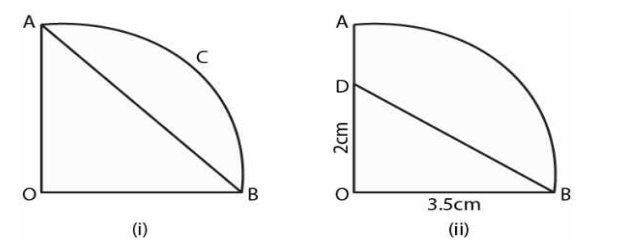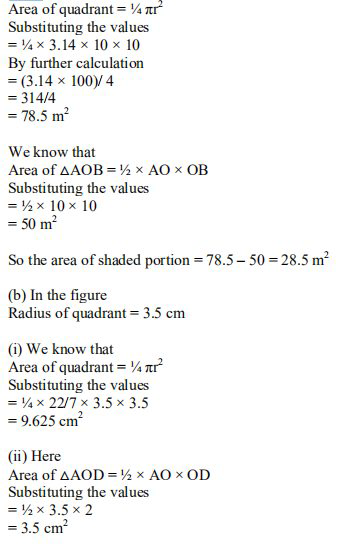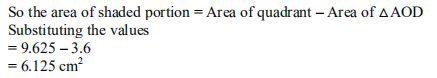# ML Aggarwal Solutions Class 9 Mathematics Solutions for Mensuration Exercise 16.3 in Chapter 16 - Mensuration

(a) In the figure (i) given below, AOBC is a quadrant of a circle of radius 10m. Calculate the area of the

(b) In the figure (ii) given below, OAB is a quadrant of a circle. The radius OA = 3.5 cm and OD = 2 cm.

Calculate the area of the shaded portion.When it comes to circles, a quarter of a circle is referred to as a quadrant, which is a 90-degree sector. When four of these quadrants are brought together, the only structure that remains is a circle.

(a) In the figure

HereRelated Questions

Lido

Courses

Teachers

Book a Demo with us

Syllabus

Maths
CBSE
Maths
ICSE
Science
CBSE

Science
ICSE
English
CBSE
English
ICSE
Coding

Terms & Policies

Selina Question Bank

Maths
Physics
Biology

Allied Question Bank

Chemistry
Connect with us on social media!